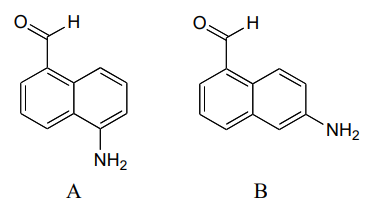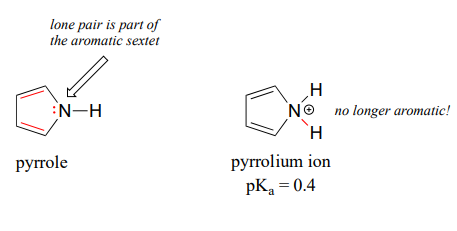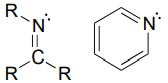# 6.6: Acid-base properties of nitrogen-containing functional groups

$$\newcommand{\vecs}{\overset { \rightharpoonup} {\mathbf{#1}} }$$ $$\newcommand{\vecd}{\overset{-\!-\!\rightharpoonup}{\vphantom{a}\smash {#1}}}$$$$\newcommand{\id}{\mathrm{id}}$$ $$\newcommand{\Span}{\mathrm{span}}$$ $$\newcommand{\kernel}{\mathrm{null}\,}$$ $$\newcommand{\range}{\mathrm{range}\,}$$ $$\newcommand{\RealPart}{\mathrm{Re}}$$ $$\newcommand{\ImaginaryPart}{\mathrm{Im}}$$ $$\newcommand{\Argument}{\mathrm{Arg}}$$ $$\newcommand{\norm}{\| #1 \|}$$ $$\newcommand{\inner}{\langle #1, #2 \rangle}$$ $$\newcommand{\Span}{\mathrm{span}}$$ $$\newcommand{\id}{\mathrm{id}}$$ $$\newcommand{\Span}{\mathrm{span}}$$ $$\newcommand{\kernel}{\mathrm{null}\,}$$ $$\newcommand{\range}{\mathrm{range}\,}$$ $$\newcommand{\RealPart}{\mathrm{Re}}$$ $$\newcommand{\ImaginaryPart}{\mathrm{Im}}$$ $$\newcommand{\Argument}{\mathrm{Arg}}$$ $$\newcommand{\norm}{\| #1 \|}$$ $$\newcommand{\inner}{\langle #1, #2 \rangle}$$ $$\newcommand{\Span}{\mathrm{span}}$$

Many of the acid-base reactions we will see throughout our study of biological organic chemistry involve functional groups which contain nitrogen. In general, a nitrogen atom with three bonds and a lone pair of electrons can potentially act as a proton-acceptor (a base) - but basicity is reduced if the lone pair electrons are stabilized somehow. We already know that amines are basic, and that the pKa for a protonated amine is in the neighborhood of 10. We also know that, due to resonance with the carbonyl bond, amide nitrogens are not basic (in fact they are very slightly acidic, with a pKa around 20).Next, let's consider the basicity of some other nitrogen-containing functional groups.

## Anilines

Aniline, the amine analog of phenol, is substantially less basic than an amine.We can use the same reasoning that we used when comparing the acidity of a phenol to that of an alcohol. In aniline, the lone pair on the nitrogen atom is stabilized by resonance with the aromatic p system, making it less available for bonding and thus less basic.Exercise $$\PageIndex{1}$$

With anilines just as with phenols, the resonance effect of the aromatic ring can be accentuated by the addition of an electron-withdrawing group, and diminished by the addition of an electron-donating group. Which of the two compounds below is expected to be more basic? Use resonance drawings to explain your reasoning.## Imines

Imines are somewhat less basic than amines: $$pK_a$$ for a protonated imine is in the neighborhood of 5-7, compared to ~10 for protonated amines. Recall that an imine functional group is characterized by an sp2-hybridized nitrogen double-bonded to a carbon.The lower basicity of imines compared to amines can be explained in the following way:

• The lone pair electrons on an imine nitrogen occupy an $$sp^2$$ hybrid orbital, while the lone pair electrons on an amine nitrogen occupy an $$sp^3$$ hybrid orbital.
• $$sp^2$$ orbitals are composed of one part $$s$$ and two parts $$p$$ atomic orbitals, meaning that they have about 33% $$s$$ character. $$sp^3$$ orbitals, conversely, are only 25% $$s$$ character (one part $$s$$, three parts $$p$$).
• An $$s$$ atomic orbital holds electrons closer to the nucleus than a $$p$$ orbital, thus $$s$$ orbitals are more electronegative than $$p$$ orbitals. Therefore, $$sp^2$$ hybrid orbitals, with their higher s-character, are more electronegative than $$sp^3$$ hybrid orbitals.
• Lone pair electrons in the more electronegative $$sp^2$$ hybrid orbitals of an imine are held more tightly to the nitrogen nucleus, and are therefore less 'free' to break away and form a bond to a proton - in other words, they are less basic.

The aromatic compound pyridine, with an imine nitrogen, has a $$pK_a$$ of 5.3. Recall from section 2.2C that the lone pair electrons on the nitrogen atom of pyridine occupy an sp2-hybrid orbital, and are not part of the aromatic sextet - thus, they are available for bonding with a proton.## Pyrrole

In the aromatic ring of pyrrole, the nitrogen lone pair electrons are part of the aromatic sextet, and are therefore much less available for forming a new bonding to a proton. Pyrrole is a very weak base: the conjugate acid is a strong acid with a $$pK_a$$ of 0.4.Below is a summary of the five common bonding arrangements for nitrogen and their relative basicity:

nitrogen group

structure

$$pK_a$$ of conjugate acid

amideNA (amide nitrogens are not basic)

amine~ 10

imine~ 5 - 7

aniline~ 5

pyrrole~ 0

Learning and being able to recognize these five different 'types' of nitrogen can be very helpful in making predictions about the reactivity of a great variety of nitrogen-containing biomolecules. The side chain of the amino acid tryptophan, for example, contains a non-basic 'pyrrole-like' nitrogen (the lone pair electrons are part of the 10-electron aromatic system), and the peptide chain nitrogen, of course, is an amide. The nucleotide base adenine contains three types of nitrogen.

.The side chain on a histidine amino acid has both a 'pyrrole-like' nitrogen and an imine nitrogen. The pKa of a protonated histidine residue is approximately 7, meaning that histidine will be present in both protonated and deprotonated forms in physiological buffer. Histidine residues in the active site of enzymes are common proton donor-acceptor groups in biochemical reactions.Exercise $$\PageIndex{2}$$

Below are the structures of four 'coenzyme' molecules necessary for human metabolism (we will study the function of all of these in chapter 17).1. When appropriate, assign a label to each nitrogen atom using the basicity classifications defined in this section ('pyrrole-like', etc.).
2. There is one nitrogen that does not fall into any of these types - is it basic? Why or why not? What would be a good two-word term to describe the group containing this nitrogen?

## Contributors

6.6: Acid-base properties of nitrogen-containing functional groups is shared under a CC BY-NC-SA license and was authored, remixed, and/or curated by Tim Soderberg.